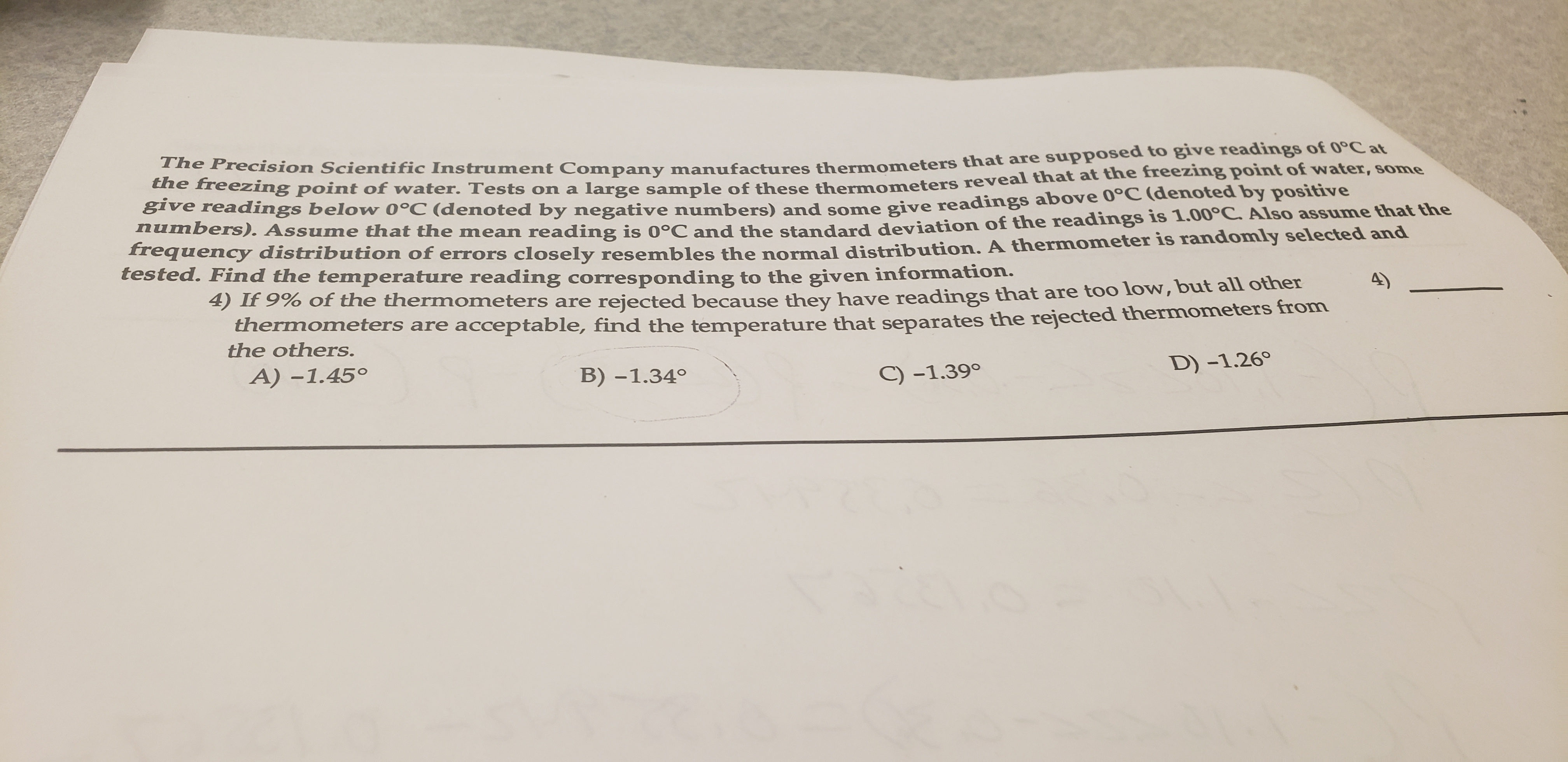# The Precision Scientific Instrument Company manufactures thermometers that are supposed to give readings of 0°Catthe freezing point of water. Tests on a large sample of these thermometers reveal that at the freezing point of water, somegive readings below 0°C (denoted by negative numbers) and some give readings above 0°C (denoted by positivenumbers). Assume that the mean reading is 0°C and the standard deviation of the readings is 1.00°C Also assume that thefrequency distribution of errors closely resembles the normal distribution. A thermometer is randomly selected andtested. Find the temperature reading corresponding to the given information.4) If 9% of the thermometers are rejected because they have readings that are too low, but all otherthermometers are acceptable, find the temperature that separates the rejected thermometers fromthe others.A) -1.450D) -1.26B) -1.34°C) -1.39°

Question

Need help with this questionhelp_outlineImage TranscriptioncloseThe Precision Scientific Instrument Company manufactures thermometers that are supposed to give readings of 0°Cat the freezing point of water. Tests on a large sample of these thermometers reveal that at the freezing point of water, some give readings below 0°C (denoted by negative numbers) and some give readings above 0°C (denoted by positive numbers). Assume that the mean reading is 0°C and the standard deviation of the readings is 1.00°C Also assume that the frequency distribution of errors closely resembles the normal distribution. A thermometer is randomly selected and tested. Find the temperature reading corresponding to the given information. 4) If 9% of the thermometers are rejected because they have readings that are too low, but all other thermometers are acceptable, find the temperature that separates the rejected thermometers from the others. A) -1.450 D) -1.26 B) -1.34° C) -1.39° fullscreen
check_circle

star
star
star
star
star
1 Rating
Step 1

It is given that the mean and standard deviation are 0 and 1, respectively.

Step 2

Let the random variable X represents the temperature.

In this case, the temperature that separates the rejected thermometers from the other represents area of 0.09 to the left of x and area of 0.91 to the right...

### Want to see the full answer?

See Solution

#### Want to see this answer and more?

Solutions are written by subject experts who are available 24/7. Questions are typically answered within 1 hour.*

See Solution
*Response times may vary by subject and question.
Tagged in

### Statistics